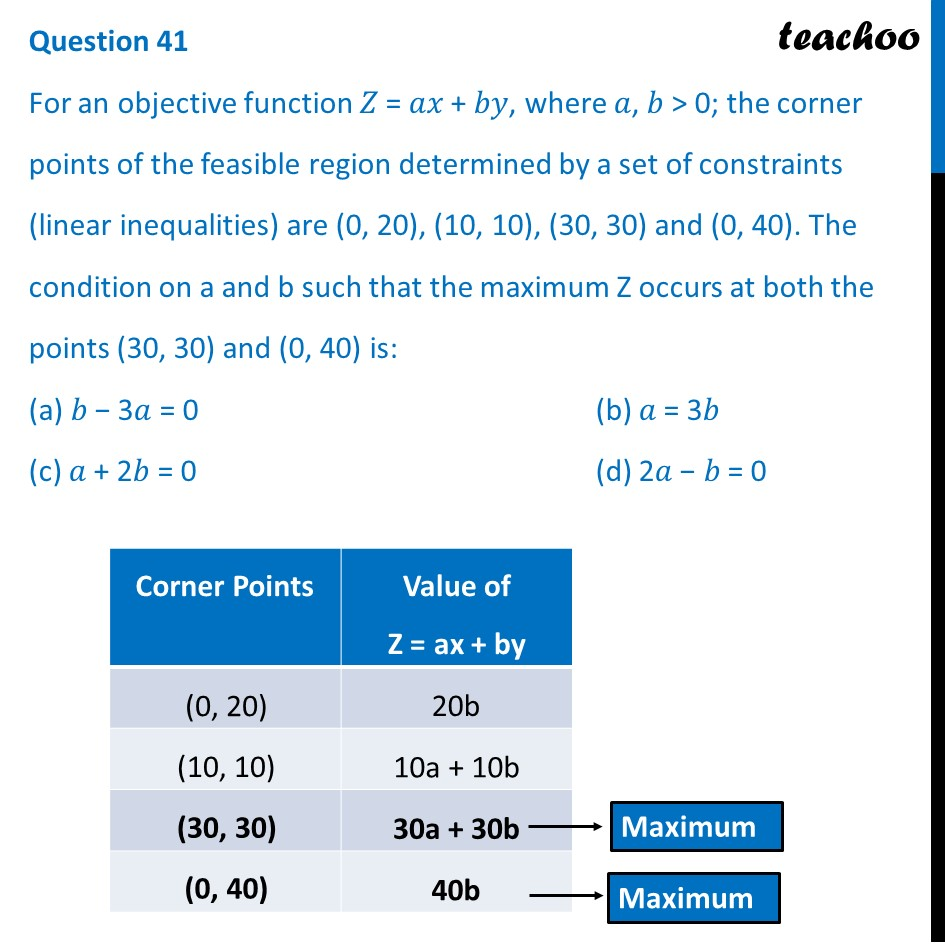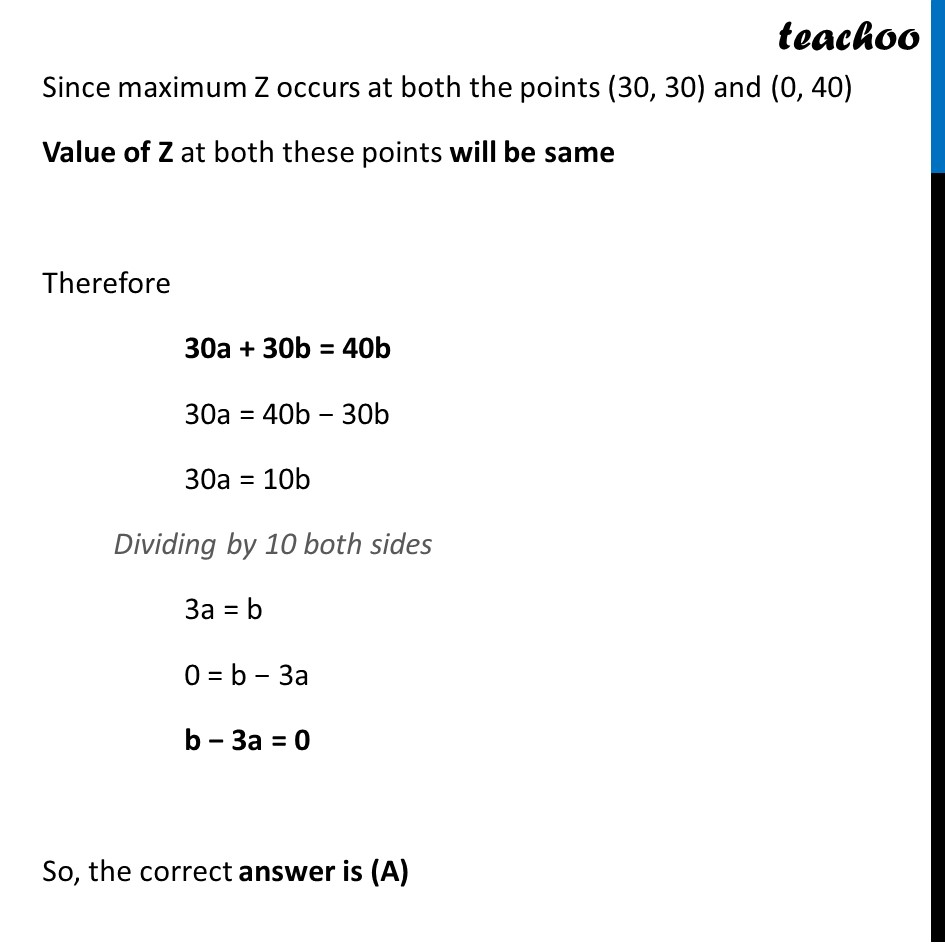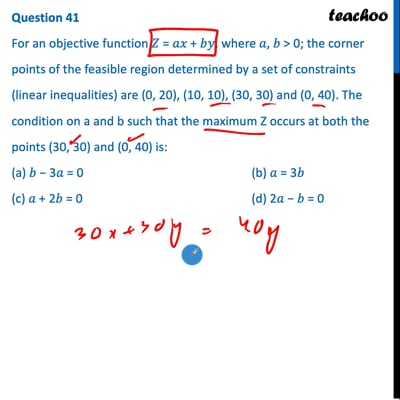CBSE Class 12 Sample Paper for 2022 Boards (MCQ Based - for Term 1)

Class 12
Solutions of Sample Papers and Past Year Papers - for Class 12 Boards

## (c) 𝑎 + 2𝑏 = 0  (d) 2𝑎 − 𝑏 = 0

This question is inspired from Ex 12.2, 11 (MCQ) - Chapter 12 Class 12 - Linear ProgrammingThis video is only available for Teachoo black users

Introducing your new favourite teacher - Teachoo Black, at only ₹83 per month

### Transcript

Question 41 For an objective function 𝑍 = 𝑎𝑥 + 𝑏𝑦, where 𝑎, 𝑏 > 0; the corner points of the feasible region determined by a set of constraints (linear inequalities) are (0, 20), (10, 10), (30, 30) and (0, 40). The condition on a and b such that the maximum Z occurs at both the points (30, 30) and (0, 40) is: (a) 𝑏 − 3𝑎 = 0 (b) 𝑎 = 3𝑏 (c) 𝑎 + 2𝑏 = 0 (d) 2𝑎 − 𝑏 = 0 Since maximum Z occurs at both the points (30, 30) and (0, 40) Value of Z at both these points will be same Therefore 30a + 30b = 40b 30a = 40b − 30b 30a = 10b Dividing by 10 both sides 3a = b 0 = b − 3a b − 3a = 0 So, the correct answer is (A)